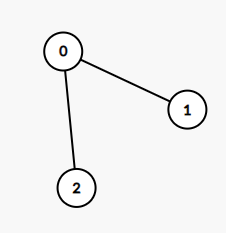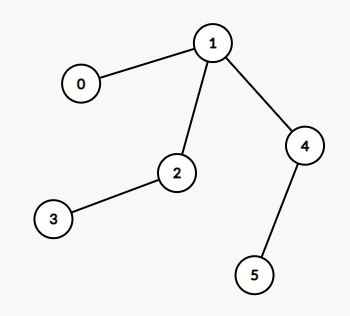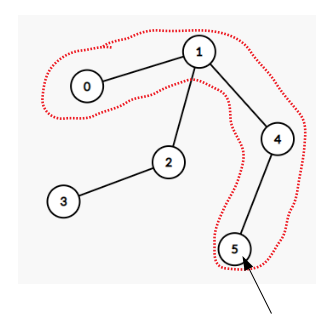Leetcode 1245：树的直径（超详细的解法！！！）
in leetcode with 0 comment输入：edges = [[0,1],[0,2]]输入：edges = [[0,1],[1,2],[2,3],[1,4],[4,5]]

• 0 <= edges.length < 10^4
• edges[i] != edges[i]
• 0 <= edges[i][j] <= edges.length
• edges 会形成一棵无向树

class Solution:
def treeDiameter(self, edges: List[List[int]]) -> int:
g, res = collections.defaultdict(list), 0
for a, b in edges:
g[a].append(b)
g[b].append(a)

def dfs(cur, pre):
nonlocal res
d1 = d2 = 0
for i in g[cur]:
if i != pre:
d = dfs(i, cur)
if d > d1:
d1, d2 = d, d1
elif d > d2:
d2 = d
res = max(res, d1 + d2)
return d1 + 1

dfs(0, None)
return resclass Solution:
def treeDiameter(self, edges: List[List[int]]) -> int:
g, n = collections.defaultdict(list), 0
for a, b in edges:
g[a].append(b)
g[b].append(a)
n = max([n, a, b])

def bfs(u):
q, dis = [u], [-1] * (n + 1)
dis[u] = 0

while q:
t = q.pop(0)
for i in g[t]:
if dis[i] == -1:
q.append(i)
dis[i] = dis[t] + 1

resDia, resId = 0, 0
for i in range(n + 1):
if dis[i] > resDia:
resDia, resId = dis[i], i
return [resId, resDia]

t1 = bfs(0)
return bfs(t1)

「如果我的文章对你有很大帮助，那么不妨～！」Responses